# Option B: Engineering Physics

See the guide for this topic.

## B.1 – Rigid bodies and rotational dynamics

• ### Torque

• When an object is acting upon by a force, it may move but it may also rotate.
• Torque is a measure of how much a force acting on an object causes that object to rotate.
• Suppose an object rotates about an axis, which we will call the pivot point ‘O’. We will call the force ‘F’. The vector distance from the pivot point to the point where the force acts is called the moment arm and is denoted by ‘r’.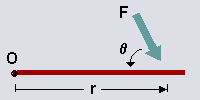• Torque may be calculated by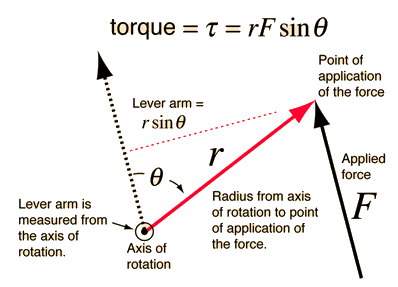• When the force ‘F’ is applied perpendicular to object (θ=90), we can remove sinθ from the equation as sin90=1.
• As torque is a vector quantity, their directions must be taken into account when calculating the resultant torque.

• ### Moment of inertia

• Just as in linear motion, mass is the tendency of a body to resist linear acceleration, the moment of inertia of an object is the tendency of a body to resist rotational acceleration.
• As torque (τ) is the rotational dynamics equivalent of force (F), moment of inertia (I) is the rotational dynamics equivalent of mass (m), the moment of inertia may be calculated bywhere I is the moment of inertia, m is the mass, and r is the vector distance from the pivot point where the force acts.

• Objects of different shapes have different moments of inertia (different tendencies of it resisting rotational acceleration) due to its mass and how the mass is distributed about the axis of rotation.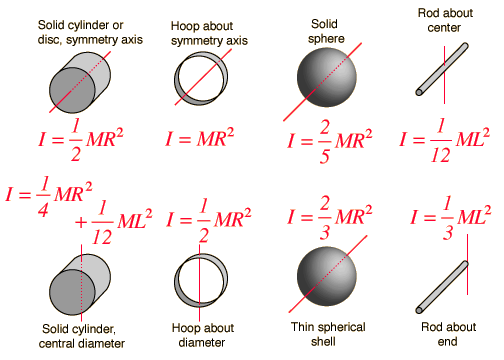Note that L is the length of the object (for cylinders and rods).

• ### Rotational and translational equilibrium

• If an object remains at rest or continues to move in exactly the same way, it is described as being in equilibrium.
• From Topic 2, we know that translational equilibrium occurs when there is no resultant force acting on an object, so that it remains stationary or continues to move with a constant velocity.
• In rotational dynamics, rotational equilibrium occurs when there is no resultant torque acting on an object, so that it remains stationary or continues to rotate with a constant angular velocity.
• To find out whether an object is in rotational and translational equilibrium, the vector sum of forces acted upon it must be calculated such as in the following example.• ### Angular acceleration

From the Topic 6 in the formula booklet, we know that angular velocity may be calculated bywhere ω is the angular velocity, f is the frequency of rotation, and T is the period of rotation.

As acceleration is the change in velocity over time, angular acceleration may be calculated by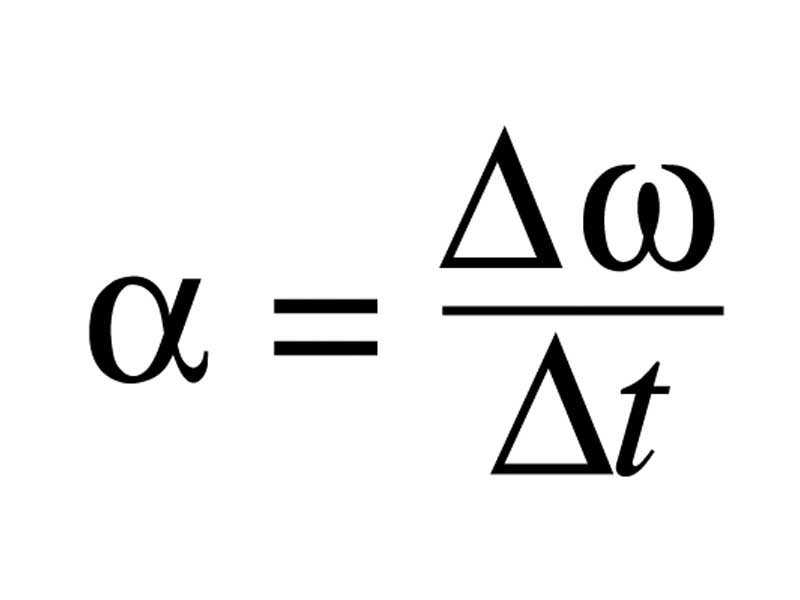where α is the angular acceleration, ω is the angular velocity, and t is time.

• ### Equations of rotational motion for uniform angular acceleration

The equations for rotational motion are very similar to those of linear motion we previously learned in Topic 2: Mechanics.• ### Newton’s second law applied to angular motion

See previous section: moment of inertia• Conservation of angular momentum
• The total angular momentum of a system is constant provided that no external torques are acting on it.
• Calculating the conservation of angular momentum is very similar to calculating the conservation of linear momentum.### FYI

Rotational kinetic energy can be calculated fromThe conservation of both linear and rotational kinetic energy is useful for calculating problems like a wheel rolling down a hill (without slipping) via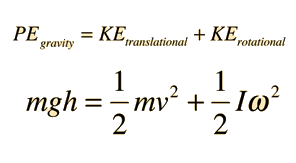## B.2 – Thermodynamics

• ### The first law of thermodynamics

The first law of thermodynamics is a statement of the principle of energy conservationwhere Q is the heat (energy) added to the system, ΔU is the change in internal energy, and W is the work done by the systems.

• ### The second law of thermodynamics

The second law of thermodynamics states that in any cyclic process, the entropy will either increase or remain the same.

This suggests that it is impossible to extract an amount of heat from a hot reservoir and use it all to do work, precluding the perfect engine.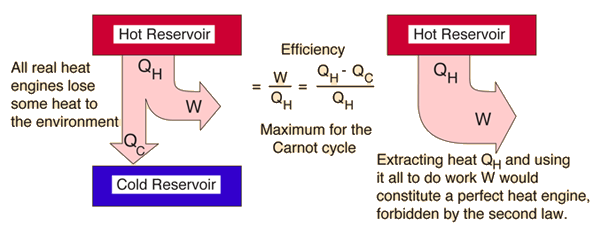This also suggests that it is not possible for heat to flow from a colder body to a warmer body without any work having been done to accomplish this flow. Energy will not flow spontaneously from a low temperature object to a higher temperature object, precluding the perfect refrigerator.• ### Entropy

Entropy in thermodynamics may be defined as a measure of the amount of energy which is unavailable to do work or a measure of the disorder of a system.

The change in entropy, ΔS, may be calculated by• ### Cyclic processes and pV diagrams

• A heat engine typically uses energy provided in the form of heat to do work and then exhausts the heat which cannot be used to do work. The first law is the application of conservation of energy to the system and the second sets limits on the possible efficiency of the machine and determines the direction of energy flow.
• Heat engines such as automobile engines operate in a cyclic manner, adding energy in the form of heat in one part of the cycle and using that energy to do useful work in another part of the cycle. This may be represented on a pV diagram.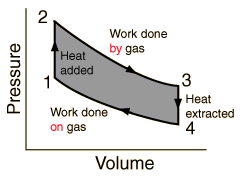• For a cyclic heat engine process, the pV diagram will be closed loop. The area inside the loop is a representation of the amount of work done during a cycle. Some idea of the relative efficiency of an engine cycle can be obtained by comparing its pV diagram with that of a Carnot cycle, the most efficient kind of heat engine cycle.

• ### Isovolumetric, isobaric, isothermal and adiabatic processes

• An isochoric or isovolumetric process is one in which the volume of the system does not change unless there is work done on or by the system. If there is no work done on or by the system then the first law of thermodynamics becomes

ΔQ=ΔU

• In an isochoric process all the thermal energy absorbed by a system goes to increase its internal energy, this usually results in an increase in temperature. An example of an isochoric process is the heating of water in a fixed volume container. As heat is added to the water the water will begin to boil, at which point the energy supplied to the system will go into vaporizing the water.
• An isobaric process is one in which the pressure of the system is constant. The heat energy added to the system does work and increases the internal energy of the system. An example could be forcing the air out of a piston slowly so that the pressure is constant throughout the piston.
• An isothermal process is one in which the temperature of the system is constant. It is possible to compress gas with a piston slowly so that the temperature of the gas itself does not change. The process is done slowly to allow the heat to transfer to the surroundings. If there is no phase change the lack of temperature change implies that there is no change in the internal energy of the gas or system. Thus we can write the first law of thermodynamics as

ΔQ=ΔW

• So all energy added to the system results in work being done by the system, or if work is done on the system heat energy leaves the system. During an isothermal process the value of pV is constant.
• An adiabatic process is one in which there is no exchange of thermal energy between a system and it surroundings (Q=0). Thus, for an adiabatic process the first law of thermodynamics becomes

ΔW=−ΔU

• In other words all the work done is at the expense of the system’s internal energy. An example of an adiabatic process is gas in an insulated piston, where the gas quickly expands and does work on the piston. This results in a decrease in internal energy and is most often accompanied by a drop in temperature.
• As no heat enters or leaves the system in an adiabatic process, it can be shown that, if the state of a fixed quantity of an ideal gas is changed from pressure p1 and volume V1 to p2 and V2,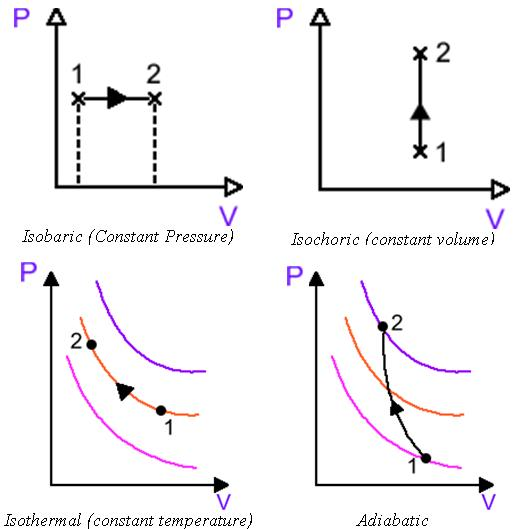The different colored lines in the bottom two diagrams represent different temperatures where a movement on a single color curve represents constant temperature and a shift across different color curves represent a shift of temperature.

### FYI

Calculating the internal energy of the system

For monatomic gases, gases which occur as single atoms such as helium and argon (in contrast to diatomic gases such as hydrogen, oxygen, and nitrogen), under ideal gas conditions, their internal energy may be given bywhere U is the internal energy of the monatomic ideal gas, n is the number of moles, R is the universal gas constant, and T is the temperature in Kelvin.

Calculating work done by the system

To calculate the work done by a gas at a non-constant pressure we must employ integration (calculus). In simple terms, integration finds the area between a curve and the x-axis. What we find is that the work done by a gas can be found from a pV diagram by finding the area under the curve.From this we can see the work done by an isobaric process is

W=pΔV

For an isochoric process the work done is

W=0

There volume does not change, no work is done, and all energy transfer is involved in internal energy or heat exchange.

For isothermal and adiabatic processes the work done is more complicated, but is still represented by the area under the curve.

• ### Carnot cycle

During one part of the cycle performed in an engine, some heat is absorbed from a hot reservoir. During another part, a smaller amount of heat is rejected to a cooler reservoir. The engine is therefore said to operate between these two reservoirs. It is a fact of experience that some heat is always rejected to the cooler reservoir.

The most efficient heat engine cycle is the Carnot cycle, consisting of two isothermal processes and two adiabatic processes. The Carnot cycle can be thought of as the most efficient heat engine cycle allowed by physical laws.

The Carnot cycle has four steps:A to B – The gas expands isothermally, while heat energy is added to the gas.
B to C – The gas expands adiabatically. Volume reaches a maximum and the pressure reaches a minimum.
C to D – The gas is compressed isothermally, while heat energy is dumped into a cold reservoir.
D to A – The gas is compressed adiabatically, Volume reaches a minimum and the pressure is maximum.• ### Thermal efficiency

The thermodynamic efficiency, η, of the engine may be defined as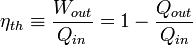where W is work done and Q is energy as W=Qin-Qout.

As the efficiency increases, the difference between the temperatures in the hot and cold reservoirs increases. At the theoretical maximum efficiency (Carnot cycle),where Tcold is the temperature in the cold reservoir and Thot is the temperature in the hot reservoir.